# Differential equation, partial, discontinuous initial (boundary) conditions

(diff) ← Older revision | Latest revision (diff) | Newer revision → (diff)

A problem involving partial differential equations in which the functions specifying the initial (boundary) conditions are not continuous.

For instance, consider the second-order hyperbolic equationand pose for it the mixed problem with initial conditions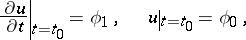and boundary conditionsIn this case the discontinuities of the initial functions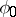and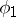entail discontinuities ofand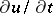along the characteristic raysand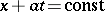, and the measure of discontinuityor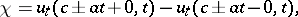whereis a discontinuity point of the functionor, satisfies the equation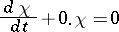along the characteristic ray, i.e.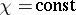. Similar results are valid for second-order hyperbolic equations with variable coefficients: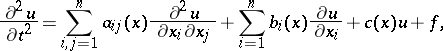In this case the discontinuities of the initial functions and the boundary conditions also entail discontinuities inandalong characteristic rays, which can be determined from the systems of equations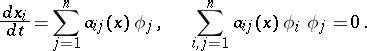The measure of discontinuitysatisfies the equation:where the function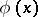defines the characteristic surface in the form of the equation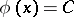.

In the case of equations of elliptic type the discontinuities of the boundary conditions do not propagate insidebecause in this case the characteristic rays are complex. For equations of elliptic type studies were made of the existence and uniqueness of the solution, and of the solution satisfying the boundary conditions. Thus, it has been proved for second-order elliptic equations in an arbitrary domain,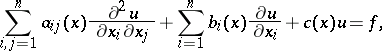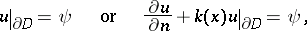that if the boundary function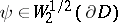for the first boundary condition and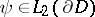for the second boundary condition, then there exists a generalized solution in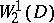which satisfies the boundary condition on the average, i.e.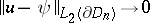, where the surfaces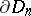approximate the surface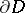. In the case of parabolic (and also elliptic) equations, the discontinuities do not propagate insideif discontinuities are present in the initial or in the boundary conditions. Problems of the existence and uniqueness of a generalized solution to the boundary condition have also been studied for these problems.

How to Cite This Entry:
Differential equation, partial, discontinuous initial (boundary) conditions. Encyclopedia of Mathematics. URL: http://encyclopediaofmath.org/index.php?title=Differential_equation,_partial,_discontinuous_initial_(boundary)_conditions&oldid=11237
This article was adapted from an original article by E.I. Moiseev (originator), which appeared in Encyclopedia of Mathematics - ISBN 1402006098. See original article